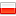### C++: Shooting yourself in the foot #5

Fri, 22 November 2019 :: #cpp :: #rant

Consider this code:

``````#include <iostream>
using namespace std;

struct Base {
};

template <typename Type>
struct Derived : Base {
Type field;
};
``````

You can see two classes here. The class `Base` contains nothing, it's a placeholder. Then, there's also class `Derived`, which contains one field. The type of this field is specified in the template parameter `Type`. So, in `main()`, let's create an instance of the `Derived` class with a field that is a `double`.

``````int main() {
Derived<double> d;
d.field = 1.23;
cout << d.field << "\n";
return 0;
}
``````

The output of this program is `1.23`, as we would expect; since we're specifying that the field of `Derived` class should be a `double`, then `cout` prints it as a double precision floating point numeric value.

Let's change our `Base` a little bit. Here's the new definition of struct `Base`:

``````struct Base {
using Type = int;
};
``````

After compiling and running the program after such change, the output value is `1`, just like we would use `Derived<int>`. But we're not using `int`, we're using `double` as a `Type` parameter. So what's happening?

Let's do an experiment! Let's perform another change to the `Base` class, like this:

``````struct Base {
using Type_XXX_HELLO_WORLD = int;
};
``````

After compiling and running the program, the result value is `1.23` again. It looks like the `using` directive inside the class shadows the template name argument in the `Derived` class. How is this possible?

Let's perform another experiment. Define the `Base` class like this:

``````template <typename NotUsed>
struct Base {
using Type = int;
};
``````

I've added a template directive here with a template parameter `NotUsed`. It's not used anywhere. The output of this program? `1.23`, as it should be.

But, if we remove the `template` definition for `Base`, the output is `1` again, which is wrong.

The bug seems to be related to shadowing the template name parameters with names declared in a non-dependent base classes. Non-dependent class means that the definition of the base class somehow depends on how we inherit from it from the parent class. In our case, the dependent class concept is introduced by using `Base<Type>` when declaring base classes for `Derived`. Non-dependent version was introduced on the very beginning of this post, where `Derived` was declared to inherit from `Base`, without any template parameters.

So, beware when inheriting from non-dependent classes. If those classes declare any type aliases, the names of those aliases can overshadow your template parameters of your derived classes. You can defend yourself from this problem by using sensible names for your template parameters.

For example, instead of using `T` as the parameter name here:

``````template <typename T>
void fun(T arg) { ... }
``````

it's better to name the template parameter according to the intention we want to use it, like:

``````template <typename Numeric>
void fun(Numeric arg) { ... }
``````

This will lessen the chance that someone uses a generic type name in the base class. Also, this would also have a benefit of allowing code reviews to take a slightly less time than before. :P

Please note that I've intentionally omitted the compiler used to compile this code. That's because all compilers suffer from this issue, and the problem is not with the compilers, but with the standard itself. There are places where C++ standard mandates how the compilation should be performed. This is one of those cases.